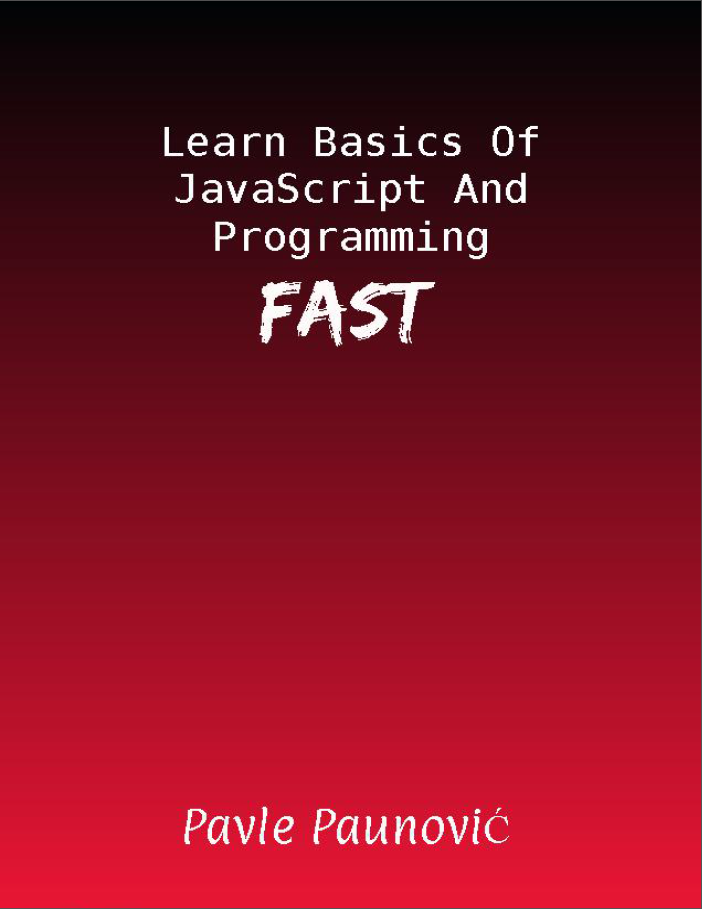# JavaScript And Programming For Beginners - Learn Basics Of Programming And JavaScript FAST

• Do you want to learn basics of JavaScript and Programming fast?
• Are you tired of 500+ pages books that leads to nowhere?
• Do you want up to date modern JavaScript and Programming guide?

## Let me show what I'm going to teach you!

### Table of content:

• Chapter 1: Basic data types
• Number
• String
• Boolean and comparison operators
• Boolean
• Comparison operators
• Logical operators
• Null and Undefined
• Comparing different data types. Arithmetic operations with different data types. Automatic
• Type Conversion
• Difference between “==”, “===” and “!=“, “!==”
• Summary
• Chapter 2: What is a variable?
• Semicolons
• Playing with basic data types and variables in console
• Declaring a variable: const vs let vs var
• Exercise : Swap the values of two variables
• Exercise solution
• Summary
• Chapter 3: Creating a .js file
• Defining multiple variables with ','
• Summary
• Chapter 4: Program control flow (If statements and let, var, const revisited)
• If-else
• Else if
• Nested if statements
• let, var, const revisited
• Exercises: Analyze following deep nested if statements
• Exercises: Analyze following deep nested if statements
• Exercises solutions
• Exercises: Scoping problem
• Exercises solutions
• Summary
• Chapter 5: Prompt, converting string to number and switch statement
• Converting string to number
• Switch statement
• Exercise: Convert if else chain to switch
• Exercise solution
• Summary
• Chapter 6: Loops
• For loops, break and continue
• Looping through string
• Nested for loops
• While loops
• Nested while loops
• Two pointer technique with while loops
• Exercise: Write nested for loops which they log to console following
• 0000 0001 0002 ….... 0999 1000 1001 ….... 9999
• Exercise solution
• Exercise : Log to console every odd number up until 50 (hint use modulo operator)
• Exercise solution
• Summary
• Chapter 7: Functions
• Function declaration, optional parameters multiple parameters
• Hoisting
• Function expression
• Anonymous function expression
• Difference between function declaration and function expression
• Arrow functions
• Scope revisited
• Closure
• Immediately Invoked Function Expression (IIFE)
• Functions as first class citizens
• Higher order functions
• Exercise: What does following returns?
• Exercises solutions
• Exercise : Make the following work
• Exercise solutions
• Summary
• Chapter 8: Arrays
• What kind of data can you put inside arrays?
• Useful methods for arrays(push, unshift, pop, shift, indexOf, splice/slice)
• Nested arrays
• Looping through arrays
• Higher Order Functions-Revisited(forEach, map, filter, reduce)
• Two pointers technique revisited
• Exercise : Duplicate [1,2,3,4] to [1,2,3,4,1,2,3,4]
• Exercise Solution
• Exercise : Find largest number in array
• Exercise solution
• Exercise : Find smallest number in array
• Exercise Solution
• Exercise : Reverse array with for loops
• Chapter 9 : Introduction To Object Oriented Programming
• Object
• Properties
• Methods
• This keyword troubles
• Class
• Encapsulation
• Module design pattern
• Inheritance
• Polymorphism
• Summary
• Chapter 10: Introduction to Functional programming
• Pure functions and side effects
• Currying
• Recursion
• Word on recursion
• Function composition
• Summary

## Get your copy today for only: 24.99\$14.99\$Learn Basics Of JavaScript And Programming Fast